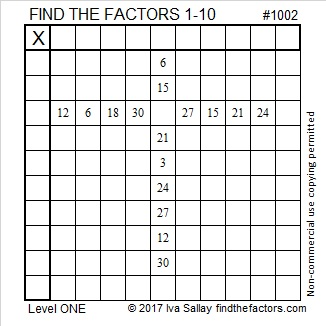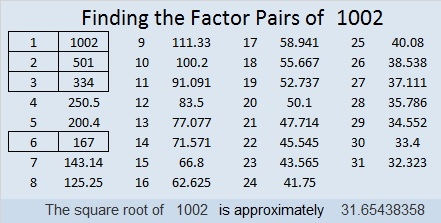# 1002 Merry Christmas!

Merry Christmas, everyone!

No matter what is going on in your life, may your day today be filled with love, peace, and joy.Print the puzzles or type the solution in this excel file: 10-factors-1002-1011

1002 is the sum of the eighteen prime numbers from 19 to 97.
It is also the sum of two consecutive prime numbers:
499 + 503 = 1002

1002 is a palindrome in two other bases:
6B6 in BASE 12 (B is 11 base 10)
2A2 in BASE 20 (A is 10 base 10)

• 1002 is a composite number.
• Prime factorization: 1002 = 2 × 3 × 167
• The exponents in the prime factorization are 1, 1, and 1. Adding one to each and multiplying we get (1 + 1)(1 + 1)(1 + 1) = 2 × 2 × 2 = 8. Therefore 1002 has exactly 8 factors.
• Factors of 1002: 1, 2, 3, 6, 167, 334, 501, 1002
• Factor pairs: 1002 = 1 × 1002, 2 × 501, 3 × 334, or 6 × 167
• 1002 has no square factors that allow its square root to be simplified. √1002 ≈ 31.65438